# 2. Summary of the book "Is Einstein Right?"

For studying of material processes of the world man artificially isolates a part of the absolute space. This isolated part is the relative space. The relative space is a measure of the absolute space and its part. The observer (man) analyzes processes of the world while being in the relative space. He has introduced to this space a measure of length for space. Space can be measured in metres, square metres, cubic metres. He has also introduced a measure of continuity – hour, day, month, year. For the analysis of the processes in the relative space he introduced a system of coordinates XYZ (Picture 1). Since all processes happen over time, man introduces a coordinate system with four dimensions. This system has terms such as the trajectory of the body’s movement, the velocity of movement etc.

Since coordinate systems are artificial and exist only in imagination, rules of usage of these systems are necessary for the analysis of the processes in nature. The absurd conclusions which contradict common sense and real processes occurring after manipulation of coordinate systems are shown in the books «IS EINSTEIN RIGHT?» and «MATTER, SPACE, TIME, GRAVITATION».

In the relative space it is necessary to analyze supposedly static coordinate systems and moving coordinate systems. The static coordinate systems are provisional, but the mocing systems are analyzed relatively to the static ones. There are no static coordinate systems in nature since there is always another external coordinate system with such properties that the first one is moving relatively to it.

Let us denote the real radial vector determining real processes in nature with the right indice 'R and the radius vector determining virtual process existing only in imagination with the left indice 'R.

Picture 1 shows a supposedly static coordinate system XYZ and moving coordinate systems X'Y'Z', X''Y''Z'', X'''Y'''Z'''.

Processes in nature can be studied relatively to a supposedly static coordinate system and relatively to coordinate systems moving relatively to each other (Picture 1).

Properties of moving bodies (velocity, trajectory etc.) relatively to a supposedly static coordinate system are described by the real vectors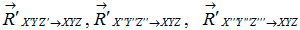(Picture 1). In the observer’s imagination real processes are formed (velocity, trajectory, time etc.) adequate to the processes in nature.

Properties of moving bodies (velocity, trajectory etc.) relatively to a moving coordinate  system are described by virtual vectors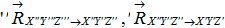(Picture 1). In the observer’s imagination virtual processes are formed (velocity, trajectory, time etc.) which do not exist in nature. They exist only in the imagination.

To receive real properties of the processes (velocity, trajectory, space, time etc.) of the world, the observer has to analyze these processes only in a chosen supposedly static coordinate system.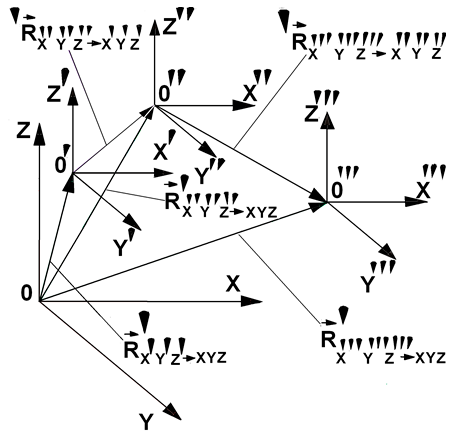Picture 1

For example, to be able to determine the real trajectories of the Sun’s movement around the center of the galaxy, the Earth’s movement around the Sun, the Moon around the Earth and also the Moon around the center of the galaxy we have to use a supposedly static coordinate system tied to the center of the galaxy (Picture 2).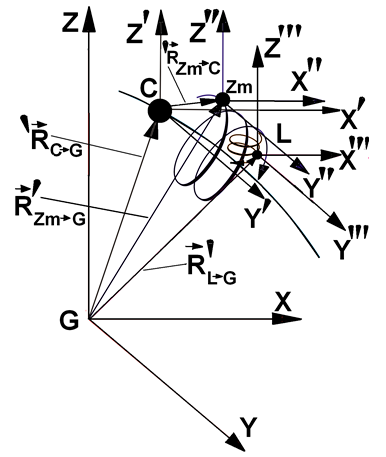Picture 2

Three coordinate systems tied to the Sun (X'Y'Z'), the Earth  (X"Y"Z") and the Moon (X'''Y'''Z'') move relatively to each other. It is impossible to determine the real movement trajectories of these macrobodies relatively to each other in one of these coordinate systems.  In the coordinate system  X"Y"Z" tied to the Earth the Sun will move around the Earth (we can see that every day). In the coordinate system X'Y'Z' tied to the Sun the Earth will move around the Sun. It is impossible to determine trajectories of the Earth and the Sun’s movement relatively to each other in one of these moving coordinate systems.

A passenger in a train cannot determine which of the two trains started moving, his or the opposite one (the two trains effect). Only after changing the coordinate system to a supposedly static (for example, tied to the station) can he understand the truth.

Only around a supposedly static coordinate system XYZ tied to the center of the galaxy  G does the Earth Zm  situated in the coordinate system X"Y"Z" move around the Sun and together with the Sun along a screw trajectory around the galactic center. The screw trajectory is described by the real radial vector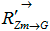.

The Moon L situated in the coordinate system X'''Y'''Z''' moves around the center of the galaxy along a double screw trajectory. One screw trajectory is observed by the Moon while moving around the Earth and with the Earth around the Sun. The second one is observed by the moon while moving with the Earth around the Sun and with the Sun around the center of the galaxy. The double screw trajectory is described by the radial vector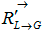(Picture 2).

The SUn С situated in the coordinate system X'Y'Z' moves around the center of the galaxy, its movement will be described by a virtual radial vectorsince the coordinate system XYZ is not external relatively to the coordinate system X'Y'Z' in which the Sun is located.

Relatively to another supposedly static coordinate system, centered in cosmos for example, the trajectories of the Sun’s, the Earth’s and the Moon’s movement will be different.

### STATEMENT 1

No experiments inside a given coordinate system can determine whether the s ystem is static or not.

### STATEMENT 2

No experiments inside a given coordinate  systems situated in one of the two coordinate systems moving relatively to each other can determine which of the two systems is moving and which is not.

The two trains effect is an example in favour of the statement 2.

### POSTULATE

To be able to determine whether n coordinate systems are moving or not and to study the properties (velocities, trajectories, times etc.) of coordinate systems moving relatively to each other, an n+1 (n 2) supposedly static coordinate system is required. The supposedly static system contains the moving coordinate systems, i.e. is external relatively to them.

The static external system is provisional because nothing is static in nature.

The books «IS EINSTEIN RIGHT» and «MATTER, SPACE, TIME, GRAVITY» study the properties of the processes (velocity, trajectories, time) in moving and supposedly static coordinate systems. It has been proven that the analysis of such properties in moving coordinate systems leads to virtual, viewable properties existing only in the imagination of the researcher. They exist only in his head and mathematical apparatus since mathematics can be used to describe any processes. It has also been proven that real properties of the processes can be received only in supposedly static coordinate systems external relatively to the moving coordinate systems and containing them.

Einstein’s relativity theory is based on the analysis of the bodies’ properties in coordinate systems moving relatively to each other, hence the conclusions derived from this theory contradict processes in nature (different time flows in coordinate systems moving relatively to each other, the dependency of velocities, lengths etc. on the coordinate system).